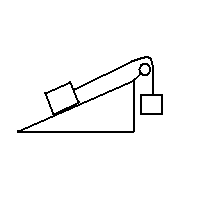# Incline plane + friction

## Homework Statement

In the figure(sorry for that quick drawing with paint) m_1 (first block) = 4.0kg, m_2 = 5.0kg and the coefficient of kinetic friction between the incline plane and the first block is \mu_k = 0.24. Incline = 30 degree with horizontal

Find the magnitude of the acceleration of the masses and the tension of the cord

## Homework Equations

(1) $$\sum Fnet = ma$$
(2) $$f_k = \mu_k*F_n$$

## The Attempt at a Solution

I applied (1) to the first block in the x-direction and then in the y-direction
Found:
$$F_n - m_1*g*\sin\theta = 0 (a_y = 0) => F_n = m_1*g*\sin\theta$$ [a]
$$T - f_k - m_1*g*\cos\theta = m_1*a_x$$

I applied (1) to block 2
$$m_2*g - T = m_2*a_y$$

$$m_2*g - f_k - m_1*g*\cos\theta = m_1*a_x + m_2*a_y$$
<=>$$m_2*g - \mu_k *m_1*g*\sin\theta - m_1*g*\cos\theta = (m_1+m_2)a$$
<=>$$g*\frac{m_2 - \mu_k *m_1*\sin\theta - m_1*\cos\theta}{m_1+m_2}=a$$

What am I doing wrong?

Here's what we got: Force from block (2) = 9.81 * 5 = 49.05N
weight of block (1) = mg = 9.81 * 4 = 39.24
Normal reaction force = 39.24cos(30) = 33.98N
Frictional force = 33.98 * 0.26 = 8.84N
force due to gravity parallel to inclined plane = 39.24sin(30) = 19.62N
Total retarding force from block (1) = 19.62N + 8.84N = 28.46N
Tension in rope = 49.05 - 28.46 = 20.60N

There is constant tension in the rope => magnitude of acceleration is constant and equal for masses.
Mass total = 4 + 5 = 9kg
a = F/m = 20.60/9.00 = 2.29ms-2

Cross my fingers I didn't read the question wrong or make any stupid mistakes. It is ridiculously late at the moment..

Your equation has gone a bit wrong..

Fn - M1*g*cos30 = 0 will be right

M2*G - T = M2*a

T - M1*G*SIN30 - FRICTIONAL FORCE = M1*A.

i think that will give the answer.

why do you have normal force in the X direction. the normal force only apply on vertical which is y direction.
BUT THIS FORMULA IS DEPENDS ON YOUR ANGLE.
so Normal force - cos(teta)mg = 0 in the y dirction
tension -mg = am

In the x direction will be sin(teta)mg = am if there is no friction .

vishal_garg, thank you so much :D I spent the whole night banging my head again my desk trying to figure out what I was doing wrong...

Thanks for all replies

No need to say that nvictor...
well are you studying in school or college.??

College :)

great...